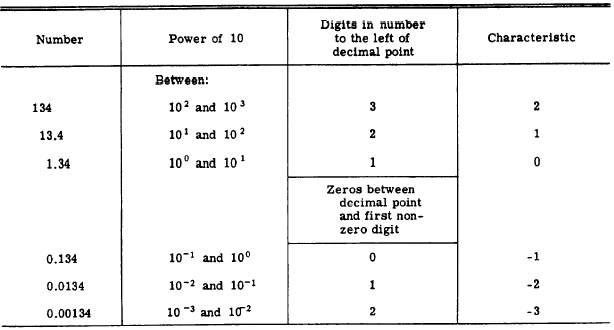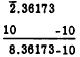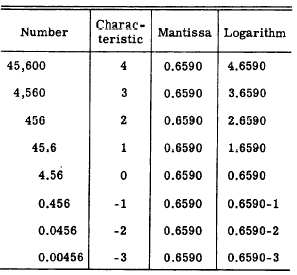Custom SearchCOMPONENTS OF LOGARITHMS The fractional part of a logarithm is usually written as a decimal. The whole number part of a logarithm and the decimal part have been given separate names because each plays a special part in relation to the number which the logarithm represents. The whole number part of a logarithm is called the CHARACTERISTIC. This part of the logarithm shows the position of the decimal point in the associated number. The decimal part of a logarithm is called the MANTISSA. For a particular sequence of digits making up a number, the mantissa of a common logarithm is always the same regardless of the position of the decimal point in that number. For example, log 5270 = 3.72181; the mantissa is 0.72181 and the characteristic is 3. CHARACTERISTIC The characteristic of a common logarithm shows the position of the decimal point in the associated number. The characteristic for a given number may be determined by inspection. It will be remembered that a common logarithm is simply an exponent of the base 10. It is the power of 10 when a number is written in scientific notation. When we write log 360 = 2.55630, we understand this to mean 10 2.55630 = 360. We know that the number is 360 and not 36 or 3,600 be cause the characteristic is 2. We know 10 is 10, 102 is 100, and 102 is 1,000. Therefore, the number whose value is 102.55630 must lie between 100 and 1,000 and of course any number in that range has 3 digits. Suppose the characteristic had been 1: where would the decimal point in the number be placed? Since 101 is 10 and 102 is 100, any number whose logarithm is between 1 and 2 must lie between 10 and 100 and will have 2 digits. Notice how the position of the decimal point changes with the value of the characteristic in the following examples: log 36,000 = 4.55630 log 3,600 = 3.55630 log 360 = 2.55630 log 36 = 1.55630 log 3.6 = 0.55630 Note that it is only the characteristic that changes when the decimal point is moved. An advantage of using the base 10 is thus revealed: If the characteristic is known, the decimal point may easily be placed. If the number is known, the characteristic may be determined by inspection; that is, by observing the location of the decimal point. Although an understanding of the relation of the characteristic to the powers of 10 is necessary for thorough comprehension of logarithms, the characteristic may be determined mechanically by application of the following rules: 1. For a number greater than 1, the characteristic is positive and is one less than the number of digits to the left of the decimal point in the number. 2. For a positive number less than 1, the characteristic is negative and has an absolute value one more than the number of zeros between the decimal point and the first nonzero digit of the number. Table 8-5 contains examples of each type of characteristic. Practice problems. In problems 1 through 4, write the characteristic of the logarithm for each number. In 5 through 8, place the decimal Table 8-5.-Positive and negative characteristics.point in each number as indicated by the characteristic (c) given for each.  1. 4,321  2. 1.23  3. 0.05  4. 12 5. 123; c = 4  6. 8,210; c = 0 7. 8; c = -1  8. 321; c = -2 Answers: 1. 3  2. 0  3. -2  4. 1 5. 12,300  6. 8.210  7. 0.8  8. 0.0321 Negative Characteristics When a characteristic is negative, such as -2, we do not carry out the subtraction, since this would involve a negative mantissa. There are several ways of indicating a negative characteristic. Mantissas as presented in the table in the appendix are always positive and the sign of the characteristic is indicated separately. For example, where log 0.023 = 2.36173, the bar over the 2 indicates that only the characteristic is negative-that is, the logarithm is -2 + 0.36173. Another way to show the negative characteristic is to place it after the mantissa. In this case we write 0.36173-2. A third method, which is used where possible throughout this chapter, is to add a certain quantity to the characteristic and to subtract the same quantity to the right of the mantissa. In the case of the example, we may write:In this way the value of the logarithm remains the same but we now have a positive characteristic as well as a positive mantissa. MANTISSA The mantissa is the decimal part of a logarithm. Tables of logarithms usually contain only mantissas since the characteristic can be readily determined as explained previously. Table 8-8 shows the characteristic, mantissa, and logarithm for several positions of the decimal point using the sequence of digits 4, 5, 6. It will be noted that the mantissa remains the same for that particular sequence of digits, regardless of the position of the decimal point. Table 8-6.-Effect of changes in the location of the decimal point.Appendix I of this training course is a table which includes the logarithms of numbers from 1 to 100. For our present purpose in using this table, we are concerned only with the first and sixth columns.  The first column contains the number and the sixth column contains its logarithm. For example, if it is desired to find the logarithm of 45, we would find the number 45 in the first column, look horizontally across the page to column 6 and read the logarithm, 1.65321. A glance down the logarithm column will reveal that the logarithms increase in value as the numbers increase in value. It must be noted in this particular table that both the mantissa and the characteristic are given for the number in the first column. This is simply an additional aid, since the characteristic can easily be determined by inspection. Suppose that we wish to use the table of Appendix I to find the logarithm of a number not shown in the "number" column. By recalling that the mantissa does not change when the decimal point moves, we may be able to determine the desired logarithm. For example, the number 450 does not appear in the number column of the table. However, the number 45 has the same mantissa as 450; the only difference between the two logs is in their .characteristics. Thus the logarithm of 450 is 2.65321. Practice problems. Find the logarithms of the following numbers: 1. 64  2. 98  3. 6400  4. 9.8 Answers: 1. 1.80618  2. 1.99123 3. 3.80618  4. 0.99123